# Difference between revisions of "12-240/Classnotes for Tuesday September 11"

In this course, we will be focusing on both a practical side and a theoretical side.

## Practical Side

1. Solving complicated systems of equations, such as: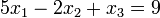$5x_1 - 2x_2 + x_3 = 9\!$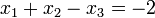$x_1 + x_2 - x_3 = -2\!$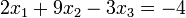$2x_1 + 9x_2 - 3x_3 = -4\!$

2. We can turn the above into a matrix!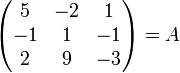$\begin{pmatrix} 5 & -2 & 1 \\ -1 & 1 & -1 \\ 2 & 9 & -3 \end{pmatrix} = A$

## Theory Side

3. "The world doesn't come with coordinates." We will learn to do all of this in a coordinate-free way.

4. We'll learn to do all of this over other sets of numbers and fields.

## Hidden Agenda

5. We'll learn the process of pure mathematics by doing it. We'll learn about:

• Abstraction
• Generalization
• Definitions
• Theorems
• Proofs
• Notation
• Logic

A number system has specific properties of the real numbers.

## Real Numbers

A set,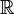$\mathbb{R}\!$, with:

• Two binary operations, addition and multiplication.
• Two special elements, 0 and 1.

The real numbers have some special properties:

### Commutative Laws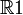$\mathbb{R}1$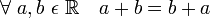$\forall\ a, b\ \epsilon\ \mathbb{R} \quad a+b = b+a\!$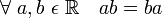$\forall\ a, b\ \epsilon\ \mathbb{R} \quad ab = ba\!$

### Associative Laws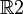$\mathbb{R}2$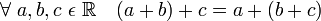$\forall\ a, b, c\ \epsilon\ \mathbb{R} \quad (a + b) + c = a + (b + c)\!$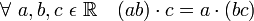$\forall\ a, b, c\ \epsilon\ \mathbb{R} \quad (ab) \cdot c = a \cdot (bc)\!$

### Existence of "Units"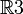$\mathbb{R}3$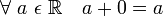$\forall\ a\ \epsilon\ \mathbb{R} \quad a + 0 = a\!$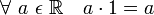$\forall\ a\ \epsilon\ \mathbb{R} \quad a \cdot 1 = a\!$

### Existence of Negatives/Inverses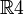$\mathbb{R}4$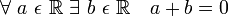$\forall\ a\ \epsilon\ \mathbb{R}\ \exists\ b\ \epsilon\ \mathbb{R} \quad a + b = 0\!$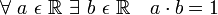$\forall\ a\ \epsilon\ \mathbb{R}\ \exists\ b\ \epsilon\ \mathbb{R} \quad a \cdot b = 1\!$

### Distributive Law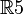$\mathbb{R}5$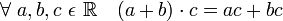$\forall\ a, b, c\ \epsilon\ \mathbb{R} \quad (a+b) \cdot c = ac + bc\!$

#### An example of a property that follows from the earlier ones: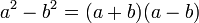$a^2 - b^2 = (a + b)(a - b)\!$

We can define subtraction and squaring from the properties covered above.

#### An example of a property that does not follow from the earlier ones:

The existence of square roots: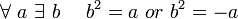$\forall\ a\ \exists\ b\ \quad b^2 = a\ or\ b^2 = -a\!$

We can construct a set that has all of the 5 properties described above, but for which this property does not follow.

This set is the rational numbers.

There is a rational number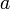$a\!$ where there is no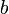$b$ in the set.

This is because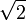$\sqrt{2}$ is irrational.

## Fields

The properties we have been discussing aren't restricted to only the real numbers.

They are also properties of:

• Rational numbers
• Complex numbers
• Others

Let's construct an abstract universe where these properties hold.

Definition: Field

• A field is a set,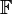$\mathbb{F}$, with:
• Two binary operations, addition and multiplication.
• Two special elements, 0 and 1, where 0 does not equal 1.
• All of the above mentioned properties hold.

Now, instead of speaking of$\mathbb{R}1,\ \mathbb{R}2,\ \mathbb{R}3,\ \mathbb{R}4,\ \mathbb{R}5$, we can speak of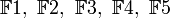$\mathbb{F}1,\ \mathbb{F}2,\ \mathbb{F}3,\ \mathbb{F}4,\ \mathbb{F}5$.

We have abstracted!

## Examples of Fields

• Take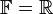$\mathbb{F} = \mathbb{R}$

• Take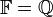$\mathbb{F} = \mathbb{Q}$ (Rational numbers)

• The complex numbers.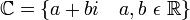$\mathbb{C} = \lbrace a + bi \quad a, b\ \epsilon\ \mathbb{R} \rbrace$

The above fields have an infinite number of elements. We can also have finite fields:

•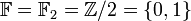$\mathbb{F} = \mathbb{F}_2 = \mathbb{Z}/2 = \lbrace 0, 1 \rbrace$
• There are only 2 elements.
• You can think of 0 as even and 1 as odd, which will help you construct the tables below.
• You can also think of the results below as the remainder of the operations when divided by 2. (mod 2)
+ 0 1
0 0 1
1 1 0
x 0 1
0 0 0
1 0 1

•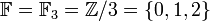$\mathbb{F} = \mathbb{F}_3 = \mathbb{Z}/3 = \lbrace 0, 1, 2 \rbrace$
+ 0 1 2
0 0 1 2
1 1 2 0
2 2 0 1
x 0 1 2
0 0 0 0
1 0 1 2
2 0 2 1

•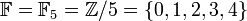$\mathbb{F} = \mathbb{F}_5 = \mathbb{Z}/5 = \lbrace 0, 1, 2, 3, 4 \rbrace$
• Not going to bother making the tables here.

•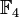$\mathbb{F}_4$ is not a field.
• It does not have the property$\mathbb{R}5$.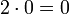$2 \cdot 0 = 0$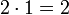$2 \cdot 1 = 2$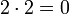$2 \cdot 2 = 0$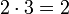$2 \cdot 3 = 2$
We never got a 1.

• If the subscript is a prime number, it is a field.

Theorem:

1.

Let F be a field.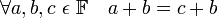$\forall a, b, c\ \epsilon\ \mathbb{F} \quad a+b = c+b$
"Cancellation Lemma"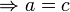$\Rightarrow a = c$

2.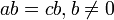$ab = cb, b \ne 0$$\Rightarrow a = c$

We'll cover 3-11 next class!

Proof of 1:

Let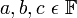$a, b, c\ \epsilon\ \mathbb{F}$
by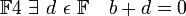$\mathbb{F} 4\ \exists\ d\ \epsilon\ \mathbb{F} \quad b+d = 0$
so with this d,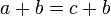$a+b = c+b\!$
and so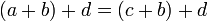$(a+b)+d = (c+b)+d\!$
so by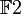$\mathbb{F} 2$,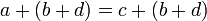$a+(b+d) = c+(b+d)\!$
so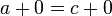$a+0 = c+0\!$
so by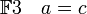$\mathbb{F} 3 \quad a = c\!$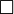$\Box$